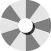• English
• German
Sale Sold Out

## Pure Paws Conditioner Oats N' Aloe 1Gallon

Out of stock
PP-OATCOND-GAL
#####################################################################################################################################################################################################...
\$148.00 \$118.40

( You save:  \$29.60)

###############################################################################################################################################################################################################################################################
Spin to win